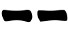## Friday, 1 July 2016

Anoher method using a single dice:
1. Roll a die;
2. Draw the line:
• If it's an even number (2,4,6), draw;
• If it's an odd number (1,3,5), draw;
3. If it's 1 or 6, it's a moving line;
4. Repeat steps 1-3 other five times drawing the hexagram from the bottom to the top.

#### Probabilities

The probabilities are similar to the three coins one but there are more chances of producing moving lines:

Prob(6) = Prob(9) = 1/6 = 16.7%
Prob(8) = Prob(7) = 2/6 = 33.3%
Prob(yin) = Prob(yang) = 1/2

#### Variations

I've found that the company Antony Publishing sells dice that works with the same principle but can directly provide the resulting line (moving lines are marked with a dot). These two images have been taken directly from their site:Single dieSet of six dice

1.Rolling 3 dice, going off odds and evens and have 3 rolls odds or 3 roll evens would result in the same changes as three coins wouldn't it?

1.Sorry the "3 roll odds and 3 roll evens" was referring to moving lines

2.Yes, if you only consider odd/even as outcome, a die becomes equivalent to a coint and, hence, using three dice this way is equivalent to using three coins!

2.I read the above article and I got some different kind of information from your article about a mattress. It is a helpful article to enhance our knowledge for us. Thankful to you for sharing an article like this. gaming stores in Leduc